﻿ 轨道车辆防滑器检测判据对防滑性能影响的仿真研究 Simulation Study on the Impact of Rail Vehicle Skid Detection Criteria on Skid Performance

Open Journal of Transportation Technologies
Vol. 09  No. 03 ( 2020 ), Article ID: 35090 , 11 pages
10.12677/OJTT.2020.93015

Simulation Study on the Impact of Rail Vehicle Skid Detection Criteria on Skid Performance

Liang Jiang, Wenliang Zhu, Mengling Wu, Yangfan Wu

Railway and Urban Rail Traffic Academy, Tongji University, Shanghai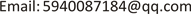Received: Mar. 25th, 2020; accepted: Apr. 10th, 2020; published: Apr. 17th, 2020ABSTRACT

Taking the train single wheel pair system as an example, a set of reasonable wheel-rail adhesion models was designed. Based on the braking distance and air consumption indexes, the slippage detection criteria of slippers were studied under low-adhesion rail surface and emergency braking conditions. The impact on the anti-skid performance of trains includes the deceleration criterion, speed difference criterion and slip rate criterion in coasting detection. The research results show that increasing the thresholds of each criterion within a reasonable range will cause the braking distance of the train to coast down and then increase, and the compressed air consumption will gradually decrease.

Keywords:Railway, WSP, Skidding Detection Criteria, Anti-Skid Performance1. 引言

2. 防滑器

$a=\frac{{S}_{低黏着}-{S}_{干轨}}{{S}_{干轨}}\text{*}100\text{%}$ (1)

${S}_{低黏着}$ 为低黏着滑行工况下的制动距离；

${S}_{干轨}$ 为干轨不滑工况下的制动距离。

$b=\frac{{V}_{低黏着}}{{V}_{干轨}}$ (2)

${V}_{低黏着}$ 为低黏着滑行工况下的耗风量，单位为NL (标升)；

${V}_{干轨}$ 为干轨不滑工况下的耗风量，单位为NL。

3. 单轮对防滑控制系统模型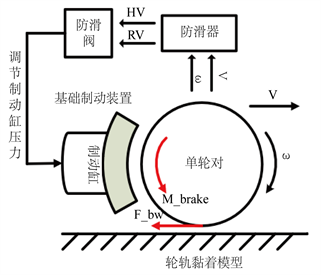Figure 1. Single wheel pair anti-skid control system model frame

$\mu =\frac{2{f}_{0}\left[\left(1-A\right){e}^{-Bw}+A\right]}{\text{π}}\left[\frac{\frac{2C\text{π}{a}^{2}b}{3Q\mu }s}{1+\left(\frac{2C\text{π}{a}^{2}b}{3Q\mu }s\right)}+\mathrm{arctan}\left(\frac{2C\text{π}{a}^{2}b}{3Q\mu }s\right)\right]$ (3)

$\mu$ 为黏着系数；

${f}_{0}$ 为轮轨最大摩擦系数；

Q为轴重；

A，B为摩擦系数调节参数；

$w$ 为相对滑动速度即 $V-\omega \cdot r$

a为轮轨椭圆接触区纵半轴长度；

b为轮轨椭圆接触区横半轴长度；

C为轮轨接触剪切刚度；

s为滑移率。Table 1. Parameters of wheel/rail adhesion calculation model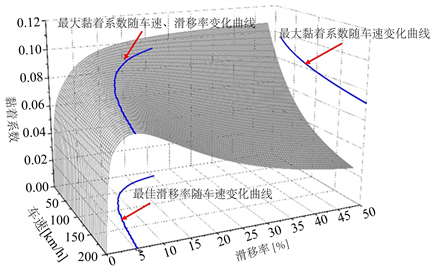Figure 2. Three-dimensional surface of adhesion coefficient, vehicle speed and slip rate under low adhesion conditions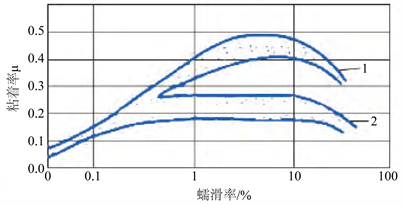1——在干燥的钢轨上；2——在潮湿的钢轨上。

Figure 3. Relationship between wheel-rail adhesion and slip ratio measured by ORE

4. 滑行检测判据对防滑性能的影响规律仿真研究Table 2. Simulation results under dry rail conditions

4.1. 减速度判据Table 3. Deceleration criterion simulation results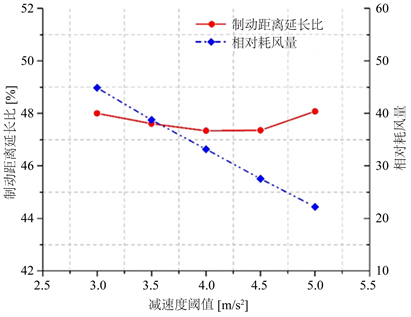Figure 4. Deceleration criterion simulation results

4.2. 速度差判据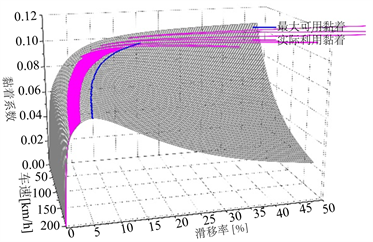(1) 减速度判据β = 3 m/s2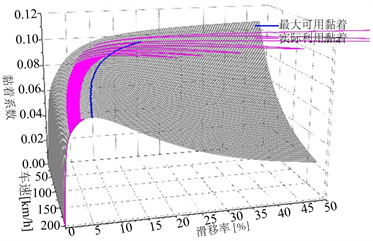(2) 减速度判据β = 3.5 m/s2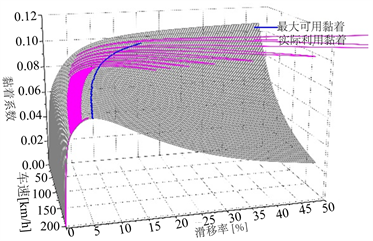(3) 减速度判据β = 4 m/s2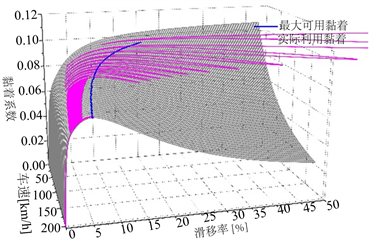(4) 减速度判据β = 4.5 m/s2(5) 减速度判据β = 5 m/s2

Figure 5. The actual use adhesion curve under different deceleration criteria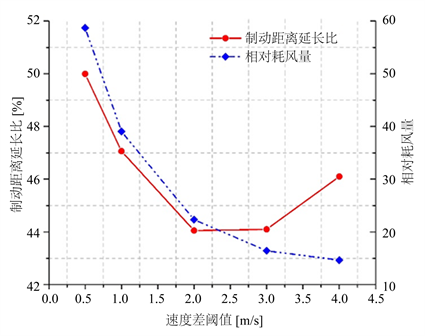Figure 6. Speed difference criterion simulation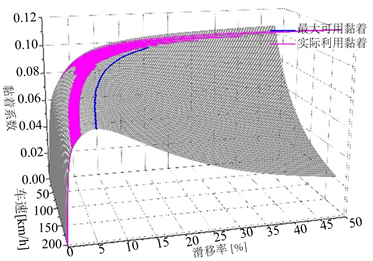(1) 速度差判据∆v = 0.5 m/s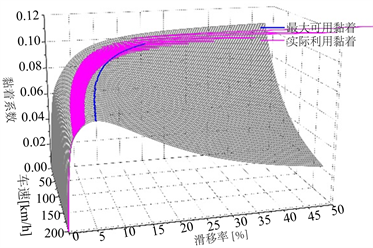(2) 速度差判据∆v = 1 m/s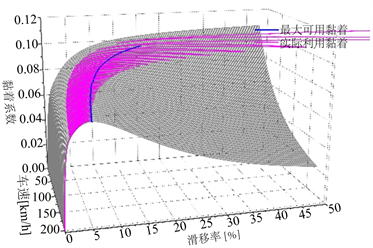(3) 速度差判据∆v = 2 m/s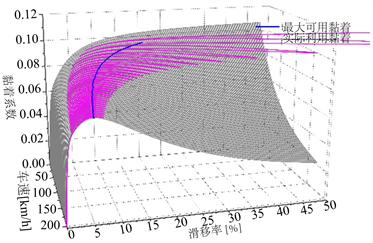(4) 速度差判据∆v = 3 m/s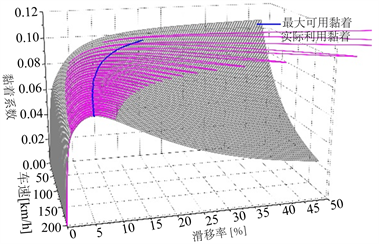(5) 速度差判据∆v = 5 m/s

Figure 7. The actual use adhesion curve under different speed difference criteria

4.3. 滑移率判据Table 5. Slip rate criterion simulation results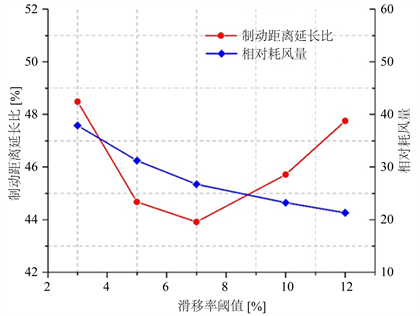Figure 8. Slip rate criterion simulation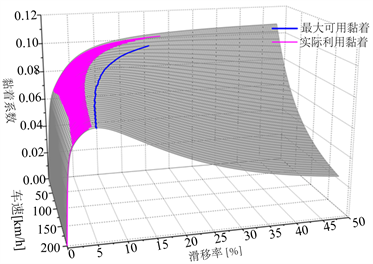(1) 滑移率判据λ = 3%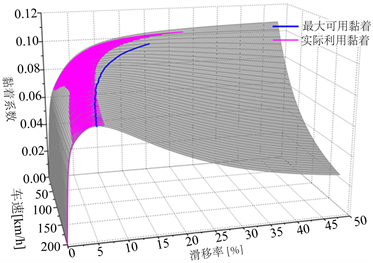(2) 滑移率判据λ = 5%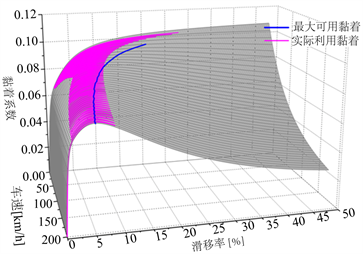(3) 滑移率判据λ = 7%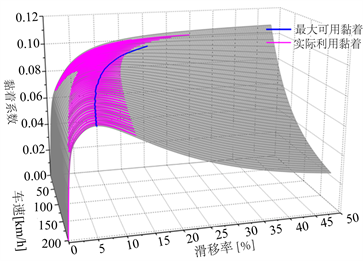(4) 滑移率判据λ = 10%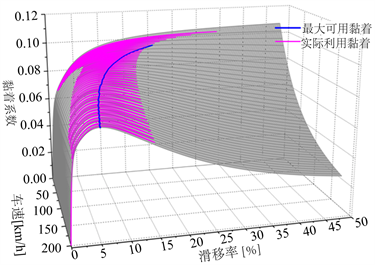(5) 滑移率判据λ = 12%

Figure 9. The actual use adhesion curve under different slip rate criteria

5. 结束语

Simulation Study on the Impact of Rail Vehicle Skid Detection Criteria on Skid Performance[J]. 交通技术, 2020, 09(03): 125-135. https://doi.org/10.12677/OJTT.2020.93015

1. 1. 饶忠. 列车制动[M]. 北京: 中国铁道出版社, 1998.

2. 2. 郭强, 车超, 赵扬宇, 马昭钰. 城市轨道交通车辆防滑性能评估标准浅析[J]. 现代城市轨道交通, 2018(5): 13-16.

3. 3. 李伟. 动车组制动系统防滑控制仿真研究[D]: [硕士学位论文]. 成都: 西南交通大学, 2011.

4. 4. 左建勇, 任利惠, 吴萌岭. 铁道车辆制动系统防滑控制仿真与试验研究[J]. 同济大学学报(自然科学版), 2010, 38(6): 912-916.

5. 5. 刁锋, 朱文良, 秦凌光, 吴萌岭. 基于有限状态机的轨道交通车辆防滑控制建模仿真与验证[J]. 城市轨道交通研究, 2019, 22(1): 55-59.

6. 6. ORE/UIC. Synthesis report: The Current State of Knowledge about Wheel-Rail Adhesion: UIC B44 RP14-1978.